# RD Sharma Solutions for Class 12 Maths Chapter 1 Relations

RD Sharma textbook for Class 12 is based on the latest syllabus prescribed by the CBSE that is the Central Board of Secondary Education. In this textbook, at the end of each chapter, an exercise consisting of multiple-choice questions and summary for quick revision of concepts and formulae have been given. In order to excel in board exams, it is suggested to refer to RD Sharma Solutions for Class 12.

Students can refer and download Chapter 1 Relations from the given links. This chapter is based on relations and types. RD Sharma Solutions cover all the topics related to it. Some of the essential topics of this chapter are listed below.

• Types of relations
• Void relation
• Universal relation
• Identity relation
• Reflexive relation
• Symmetric relation
• Transitive relation
• Antisymmetric relation
• Equivalence relation
• Theorems based on relations

## Download the PDF of RD Sharma Solutions For Class 12 Maths Chapter 1 Relations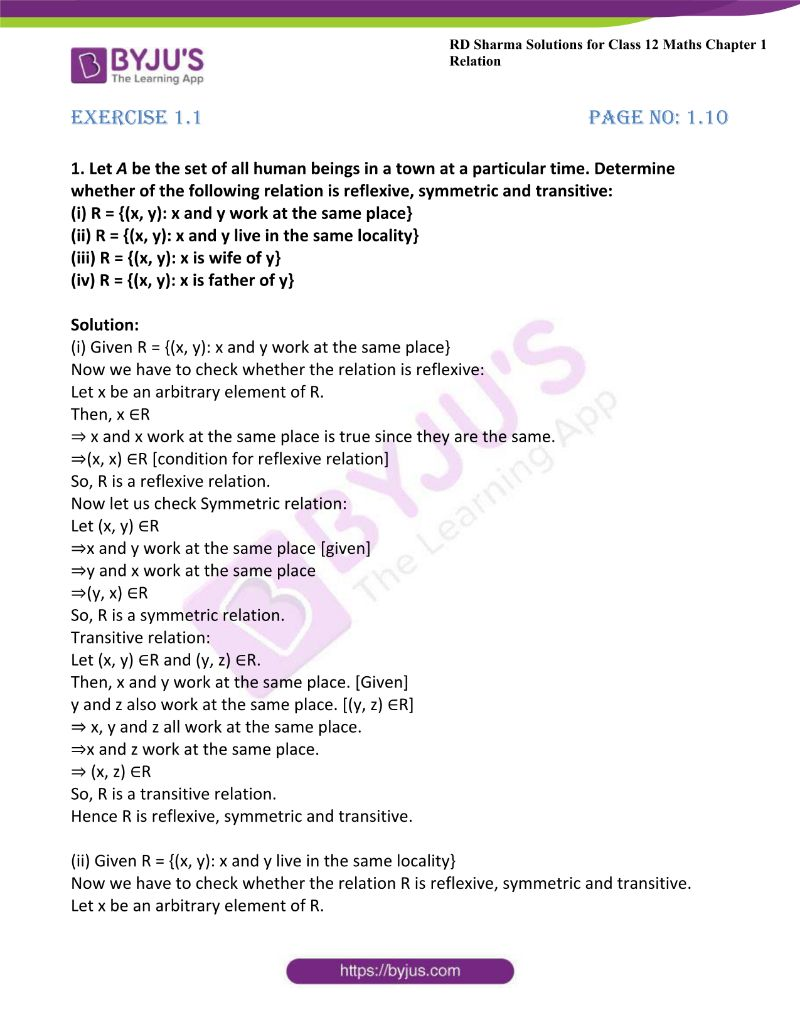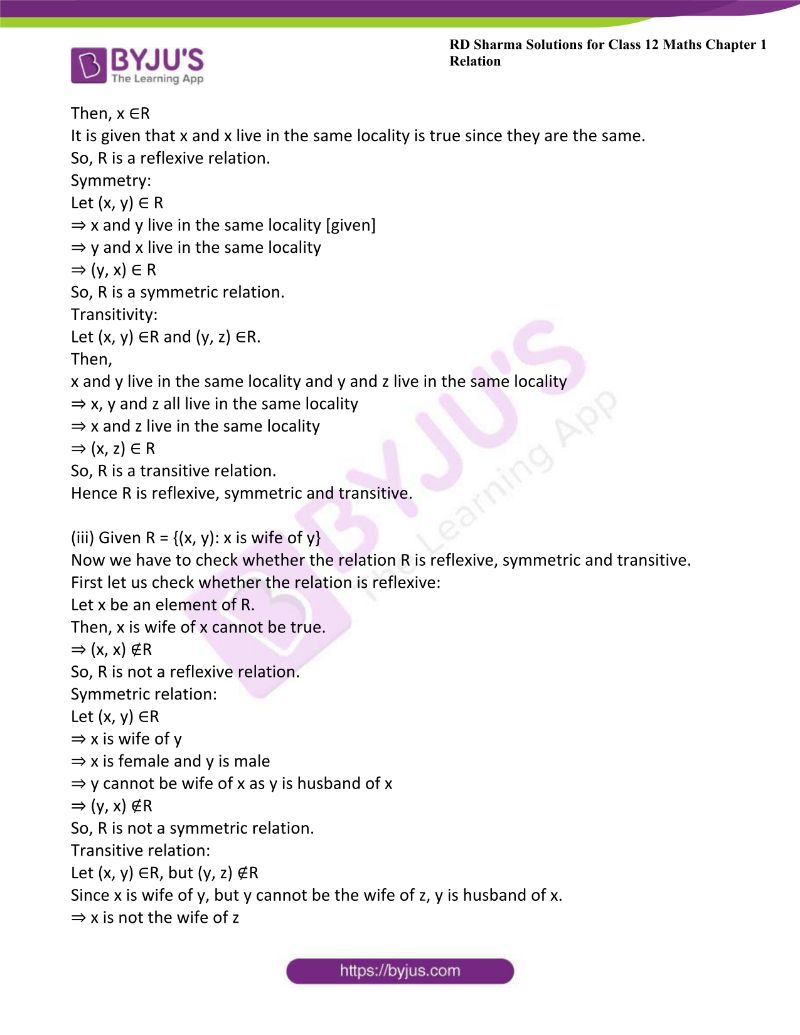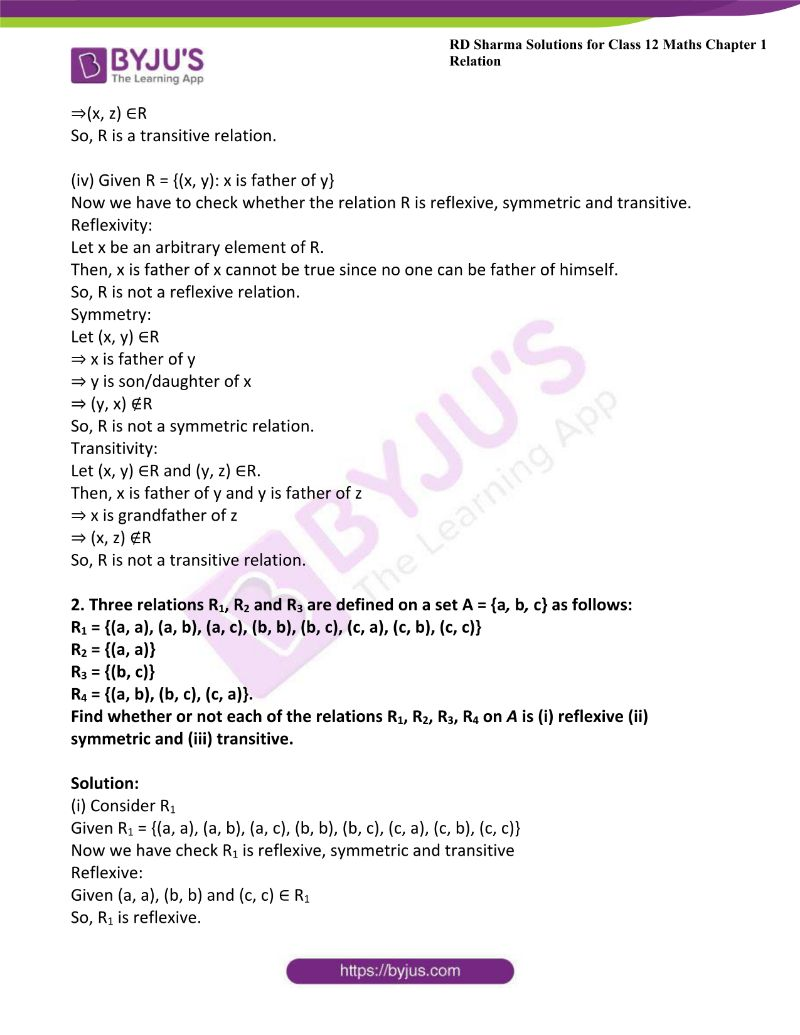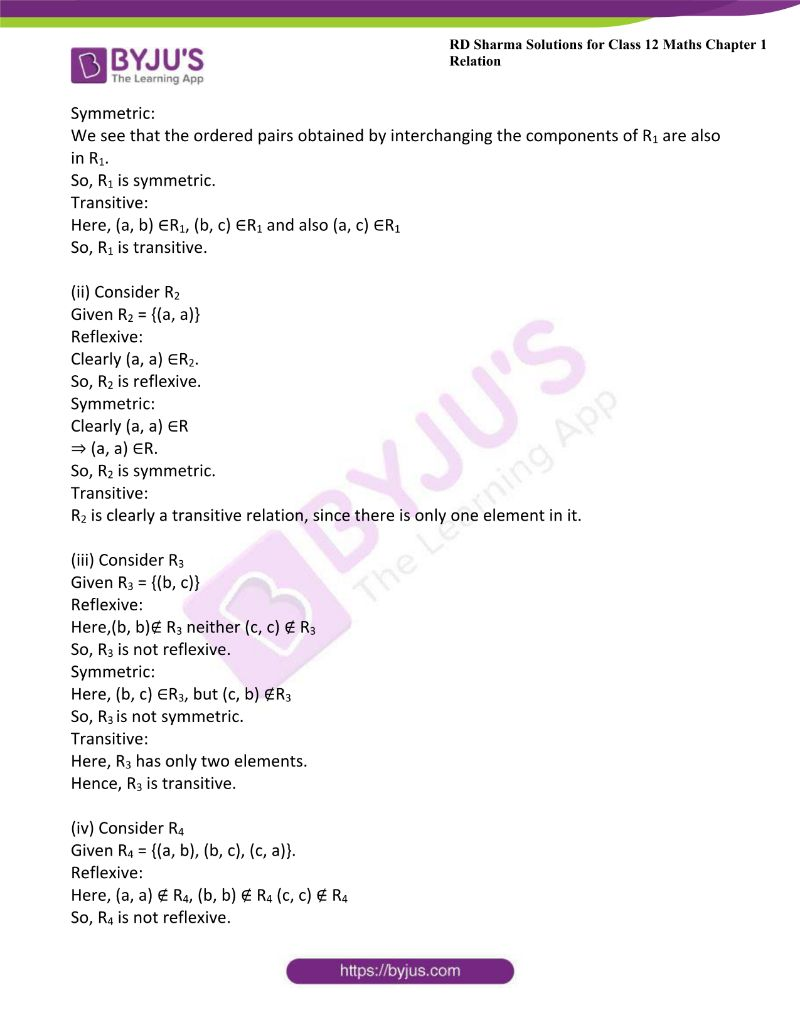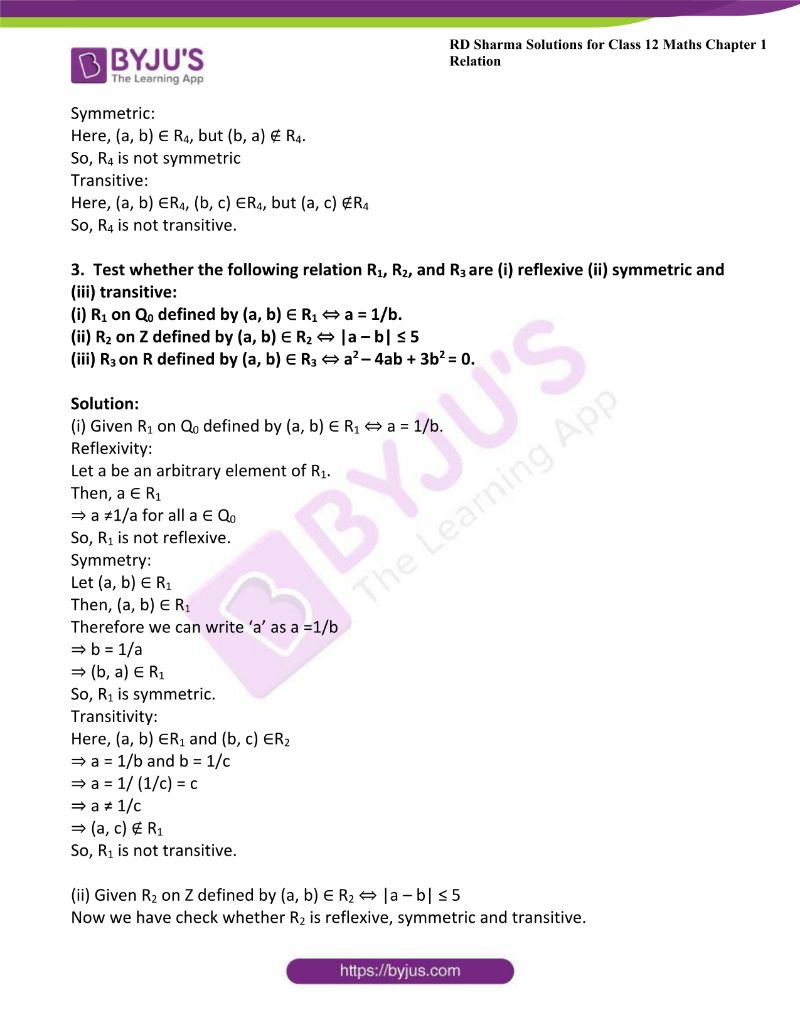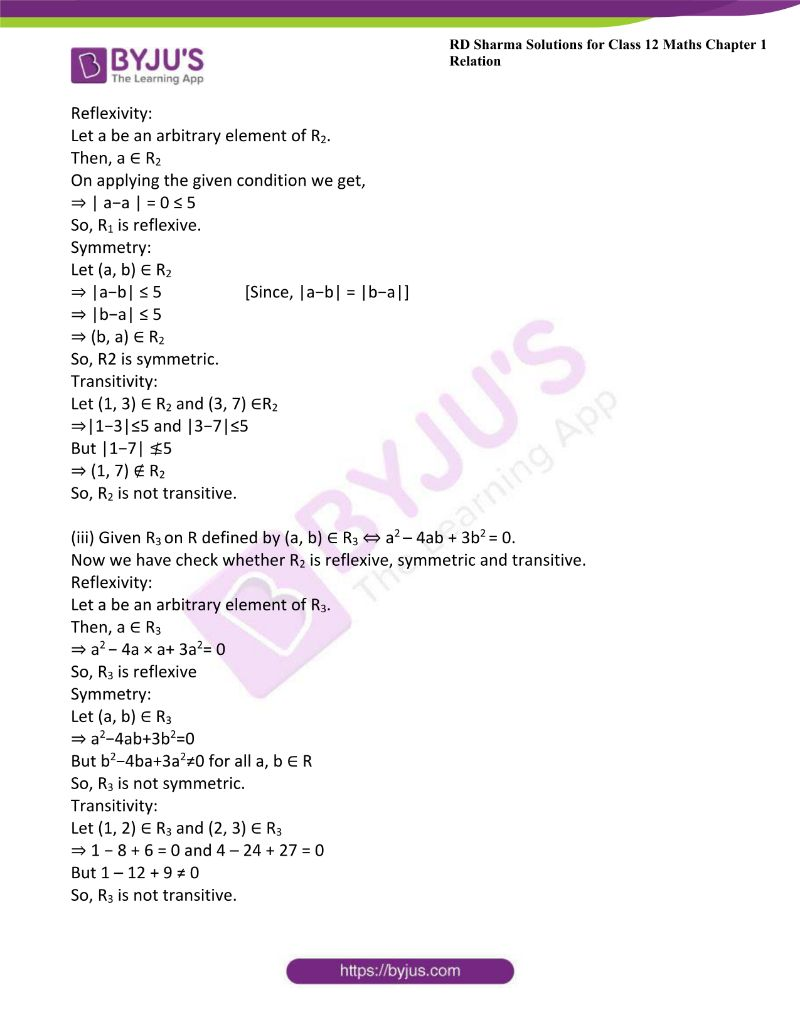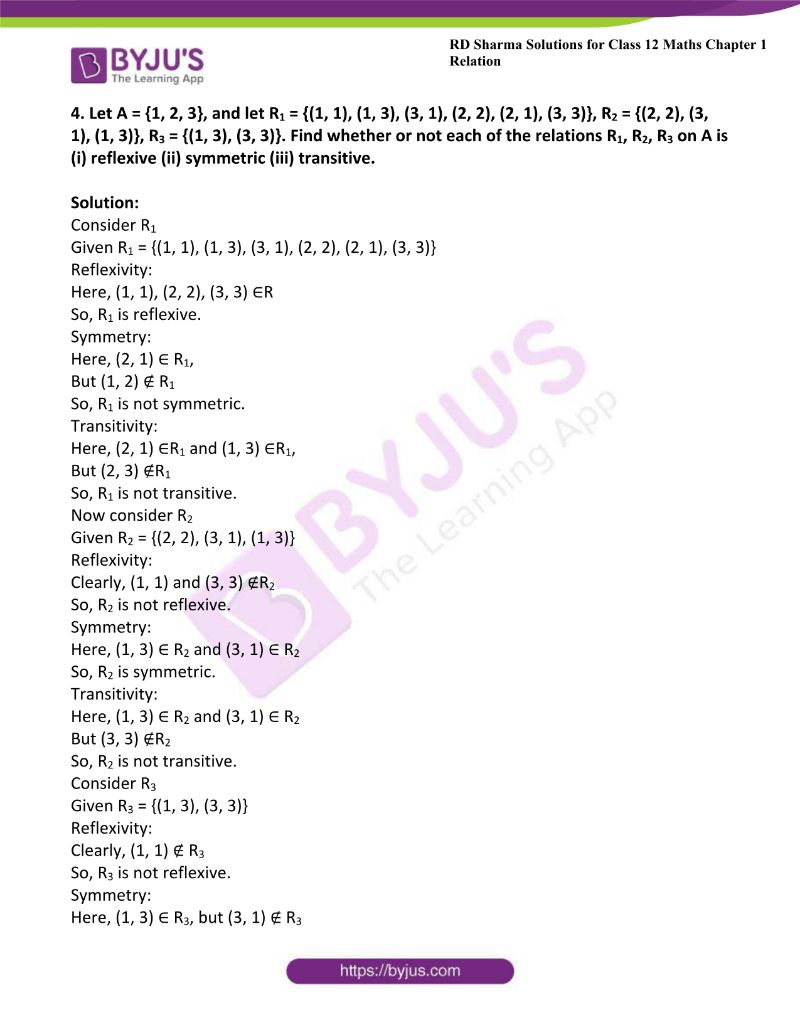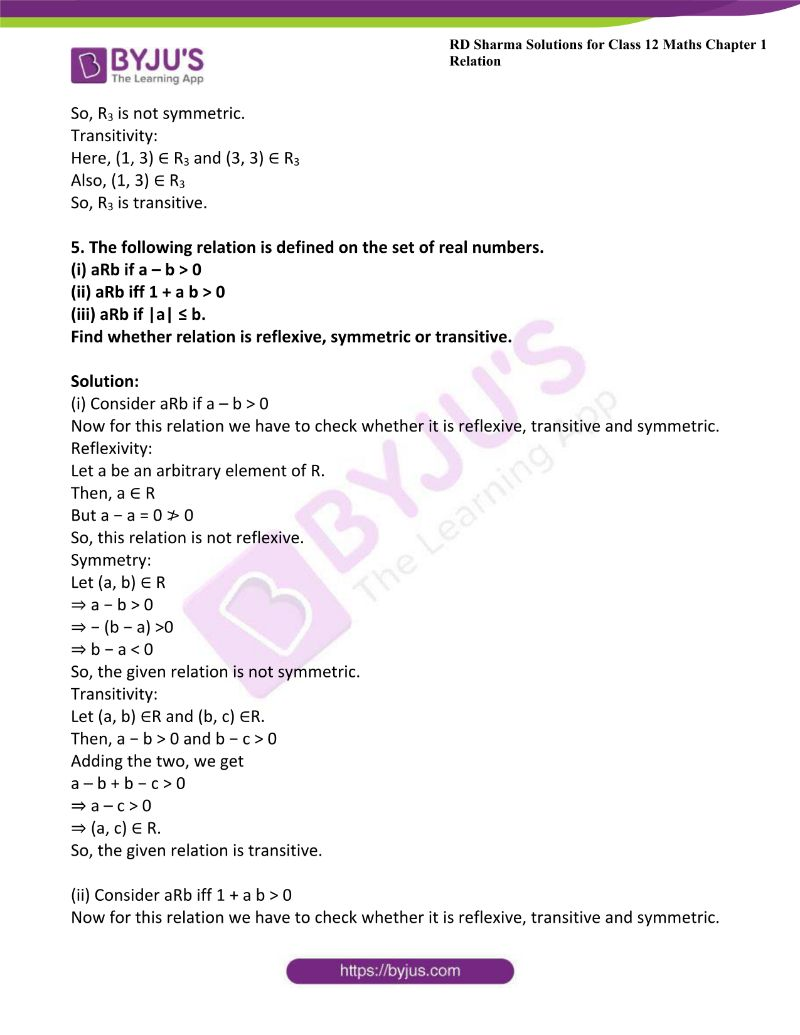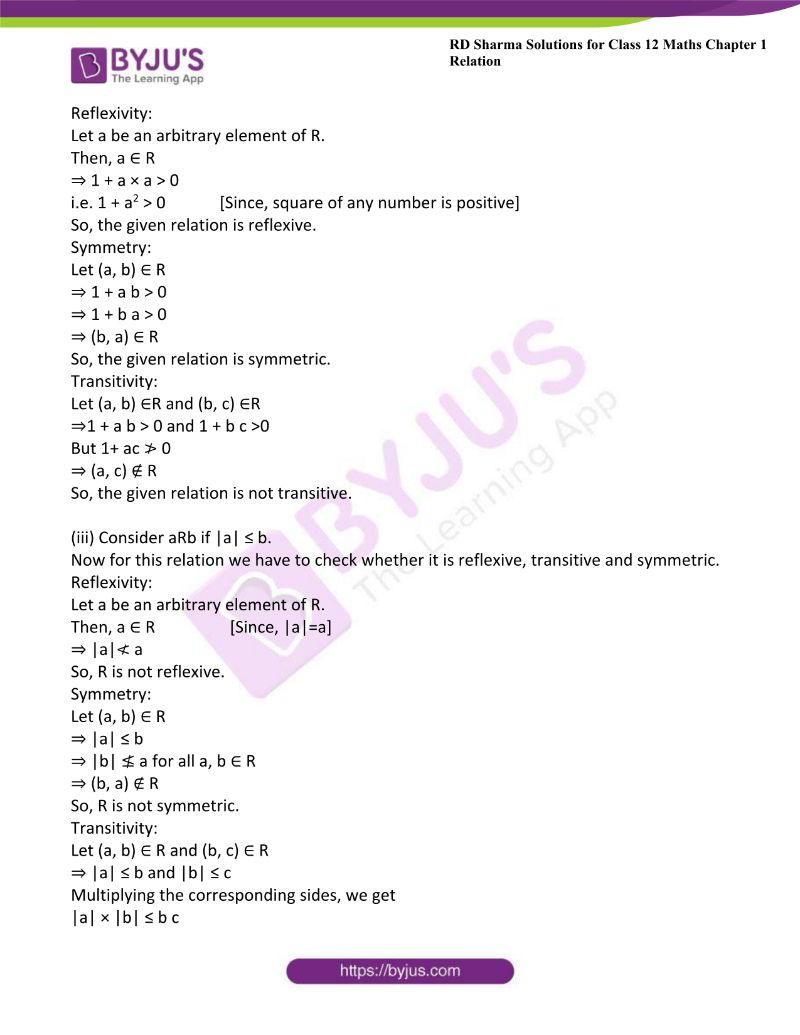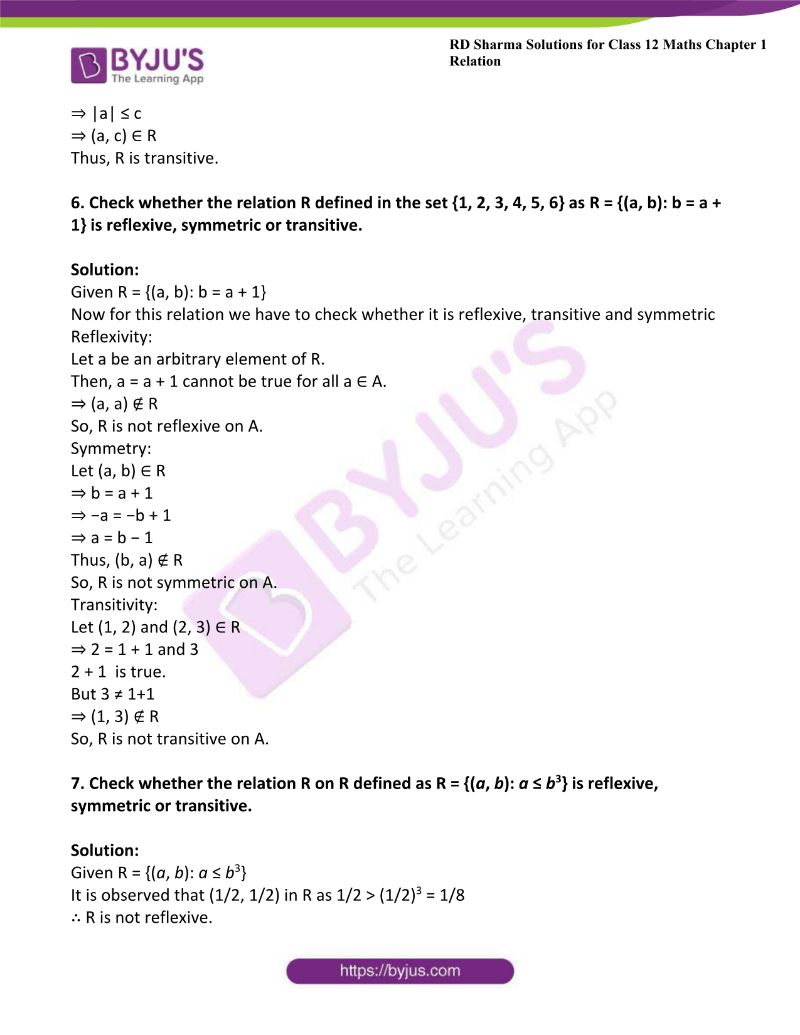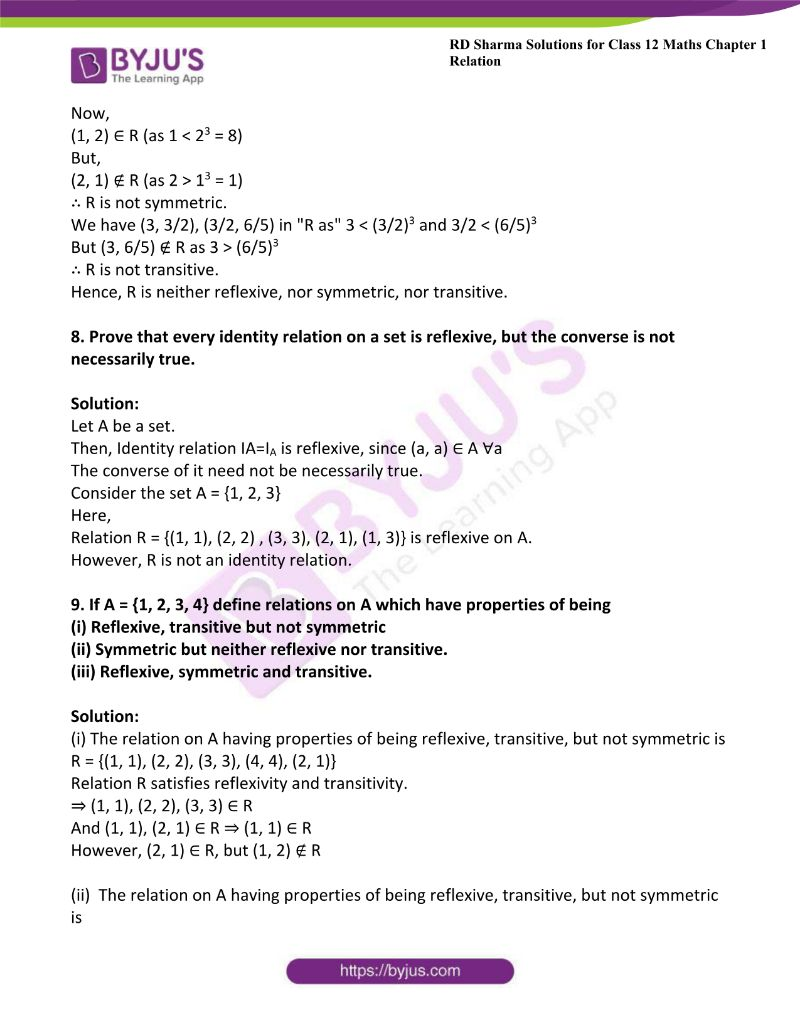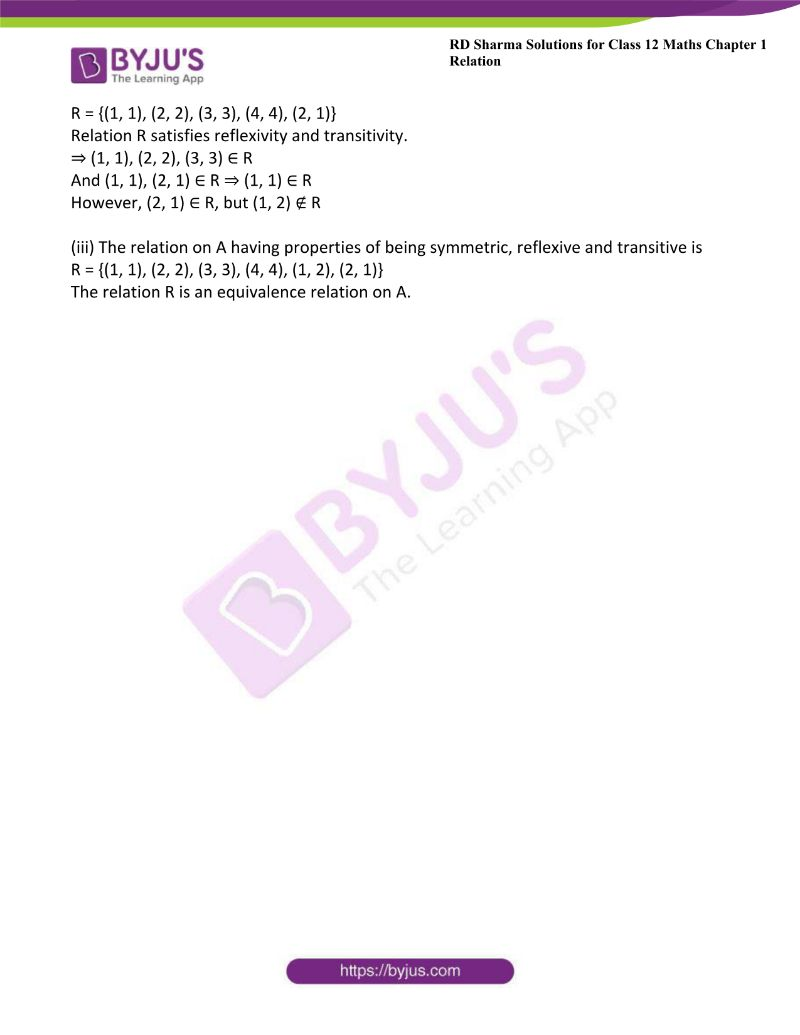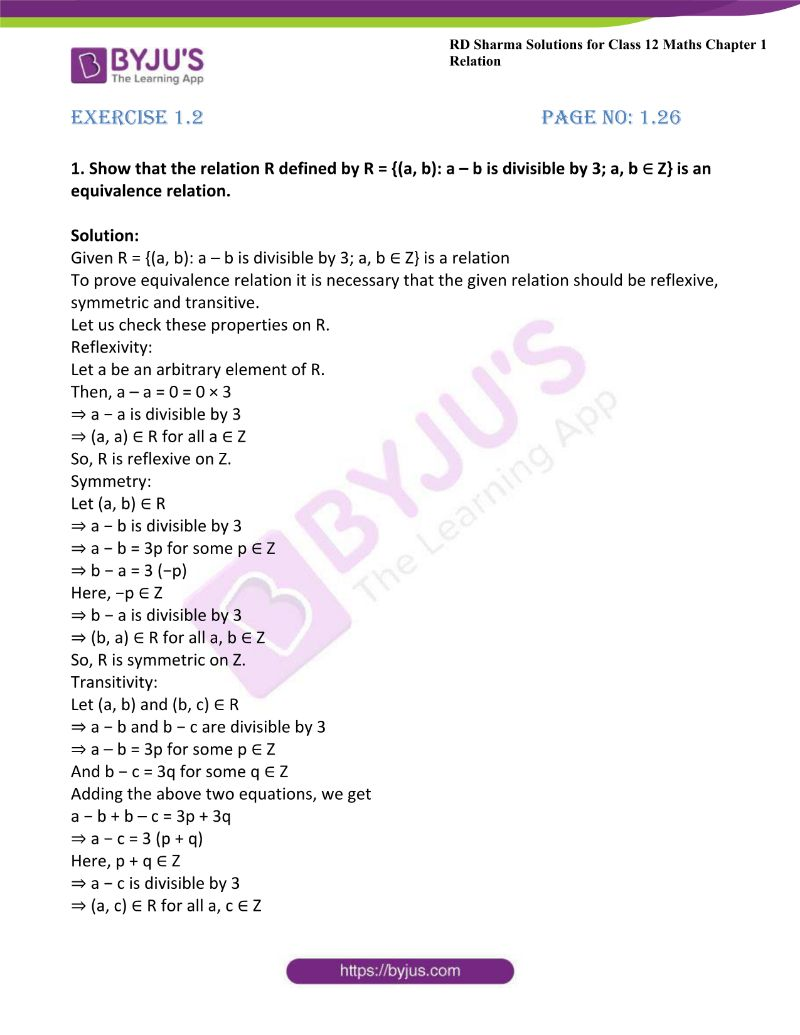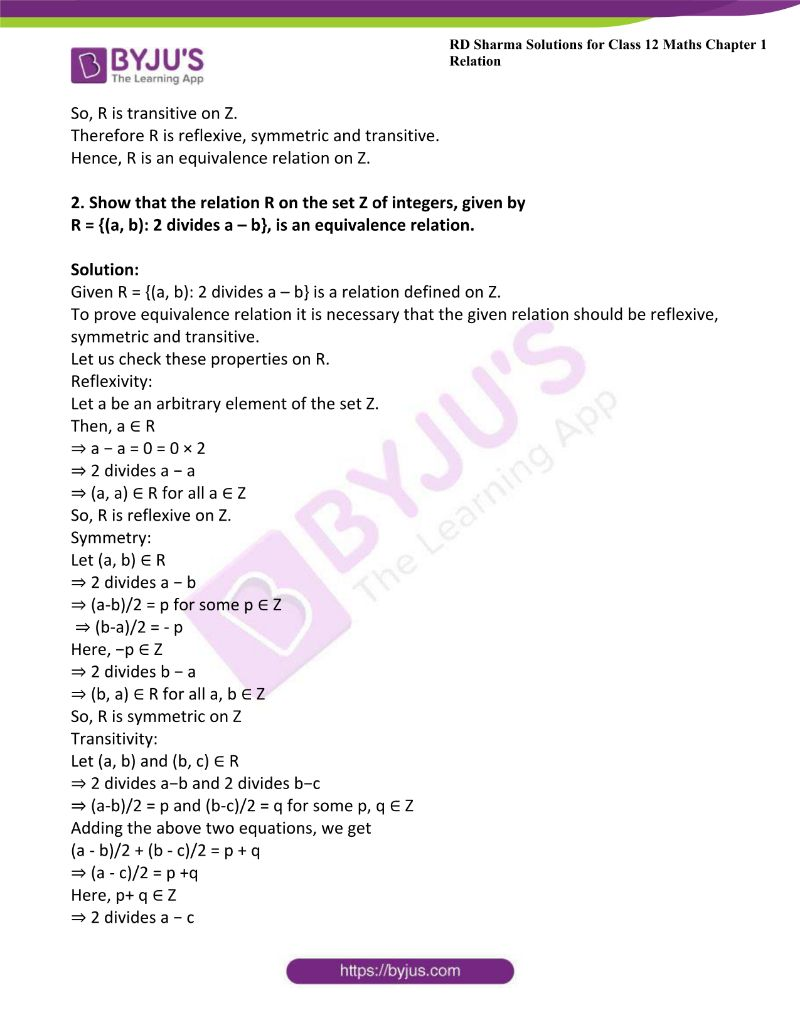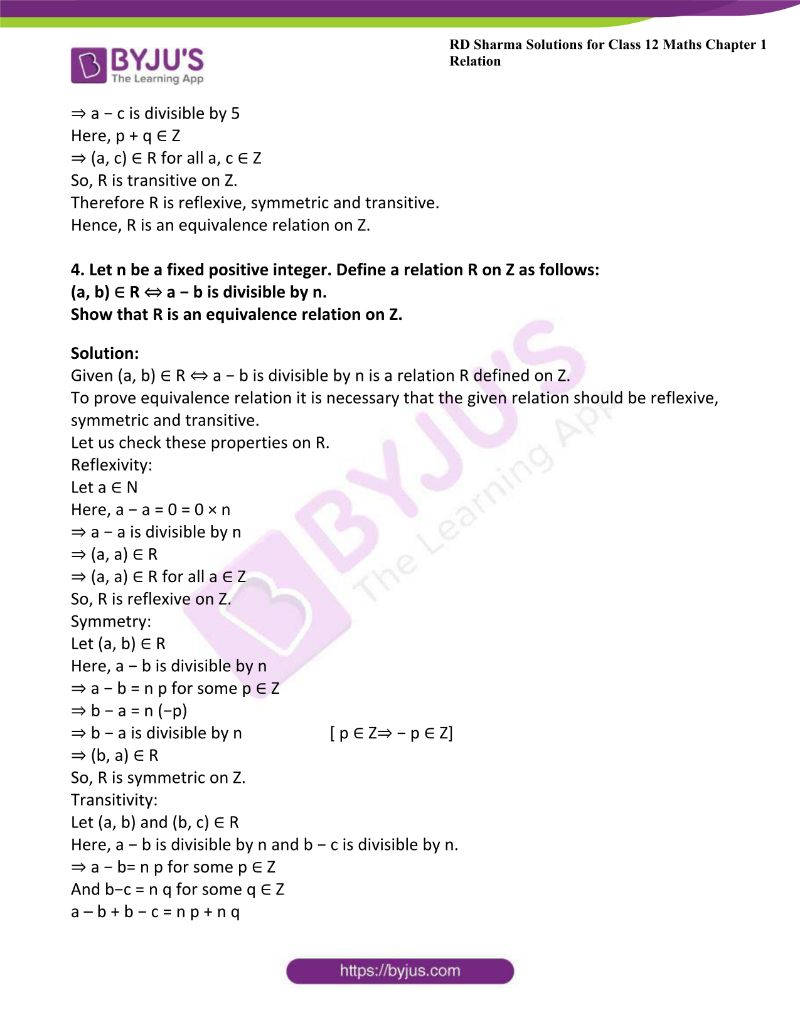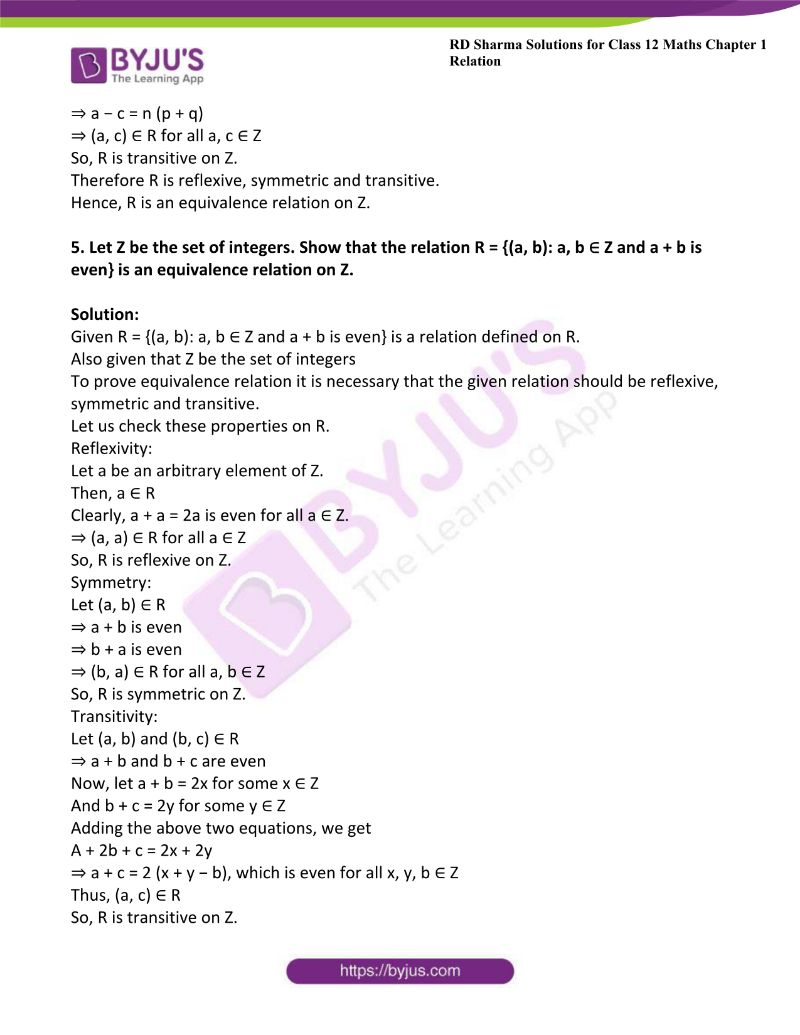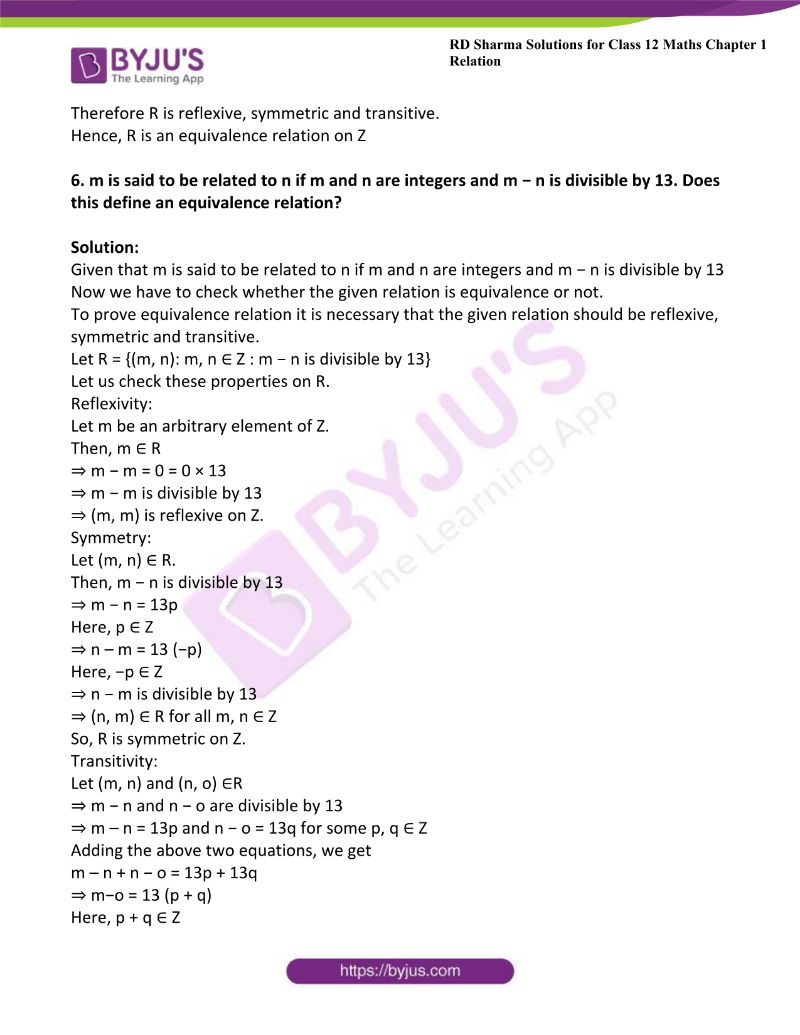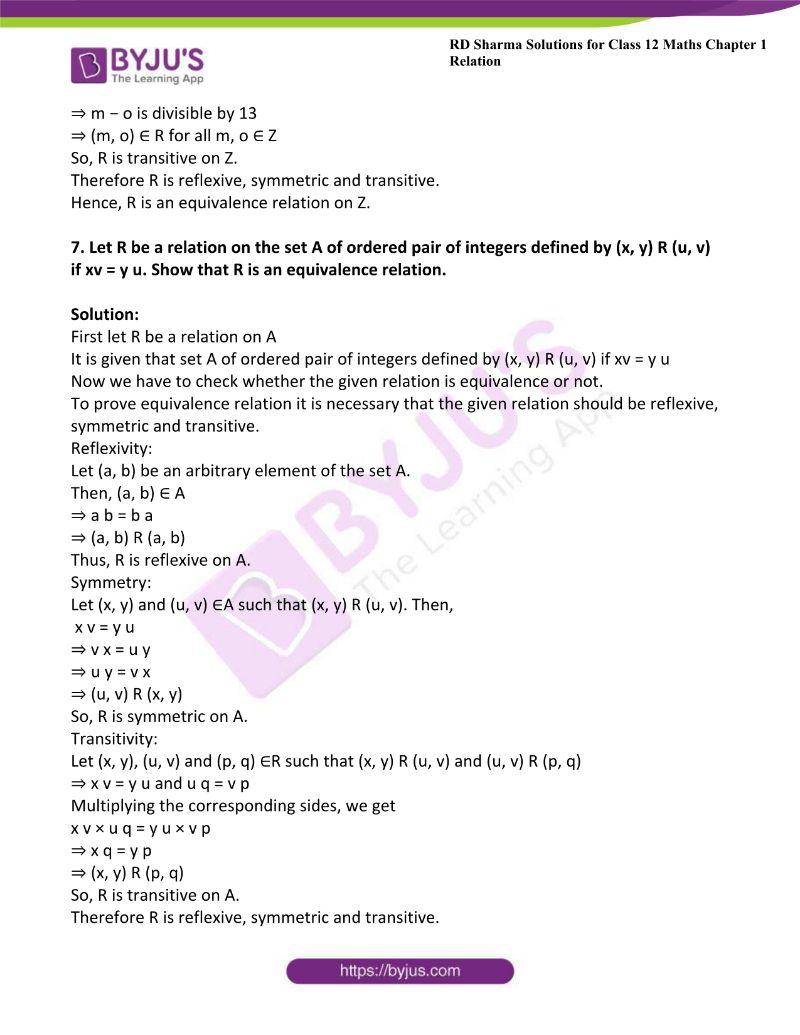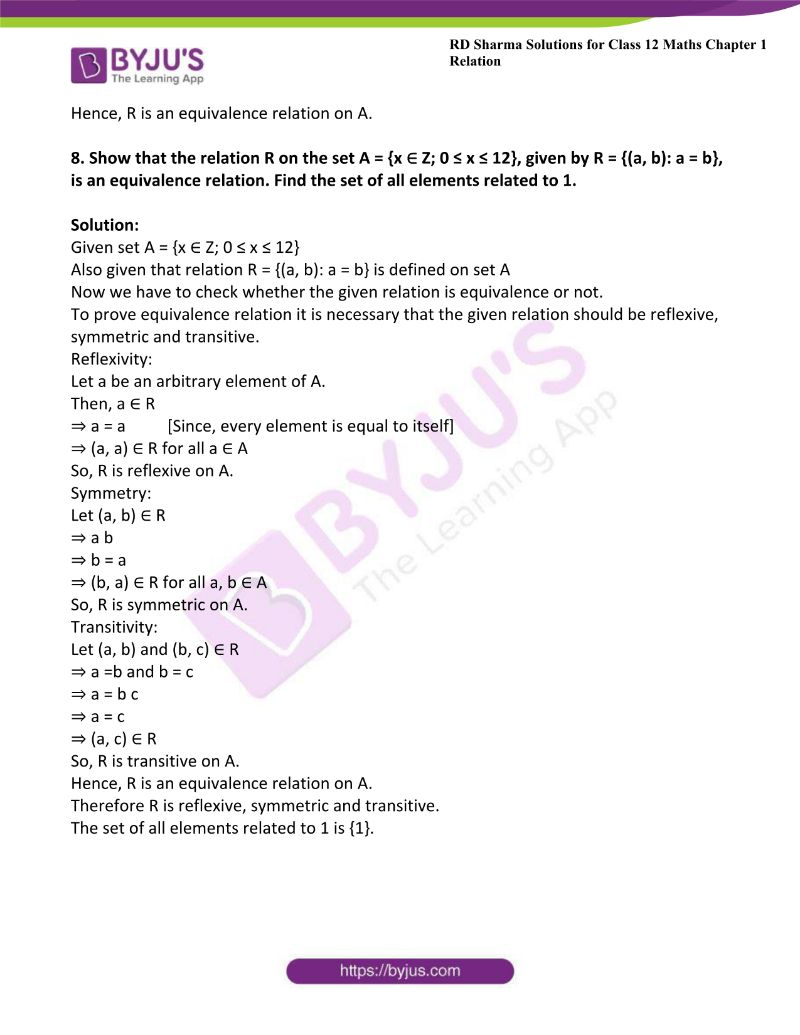### Access answers to Maths RD Sharma Solutions For Class 12 Chapter 1 – Relations

Exercise 1.1 Page No: 1.10

1. Let A be the set of all human beings in a town at a particular time. Determine whether of the following relation is reflexive, symmetric and transitive:

(i) R = {(x, y): x and y work at the same place}

(ii) R = {(x, y): x and y live in the same locality}

(iii) R = {(x, y): x is wife of y}

(iv) R = {(x, y): x is father of y}

Solution:

(i) Given R = {(x, y): x and y work at the same place}

Now we have to check whether the relation is reflexive:

Let x be an arbitrary element of R.

Then, x ∈R

⇒ x and x work at the same place is true since they are the same.

⇒(x, x) ∈R [condition for reflexive relation]

So, R is a reflexive relation.

Now let us check Symmetric relation:

Let (x, y) ∈R

⇒x and y work at the same place [given]

⇒y and x work at the same place

⇒(y, x) ∈R

So, R is a symmetric relation.

Transitive relation:

Let (x, y) ∈R and (y, z) ∈R.

Then, x and y work at the same place. [Given]

y and z also work at the same place. [(y, z) ∈R]

⇒ x, y and z all work at the same place.

⇒x and z work at the same place.

⇒ (x, z) ∈R

So, R is a transitive relation.

Hence R is reflexive, symmetric and transitive.

(ii) Given R = {(x, y): x and y live in the same locality}

Now we have to check whether the relation R is reflexive, symmetric and transitive.

Let x be an arbitrary element of R.

Then, x ∈R

It is given that x and x live in the same locality is true since they are the same.

So, R is a reflexive relation.

Symmetry:

Let (x, y) ∈ R

⇒ x and y live in the same locality [given]

⇒ y and x live in the same locality

⇒ (y, x) ∈ R

So, R is a symmetric relation.

Transitivity:

Let (x, y) ∈R and (y, z) ∈R.

Then,

x and y live in the same locality and y and z live in the same locality

⇒ x, y and z all live in the same locality

⇒ x and z live in the same locality

⇒ (x, z) ∈ R

So, R is a transitive relation.

Hence R is reflexive, symmetric and transitive.

(iii) Given R = {(x, y): x is wife of y}

Now we have to check whether the relation R is reflexive, symmetric and transitive.

First let us check whether the relation is reflexive:

Let x be an element of R.

Then, x is wife of x cannot be true.

⇒ (x, x) ∉R

So, R is not a reflexive relation.

Symmetric relation:

Let (x, y) ∈R

⇒ x is wife of y

⇒ x is female and y is male

⇒ y cannot be wife of x as y is husband of x

⇒ (y, x) ∉R

So, R is not a symmetric relation.

Transitive relation:

Let (x, y) ∈R, but (y, z) ∉R

Since x is wife of y, but y cannot be the wife of z, y is husband of x.

⇒ x is not the wife of z

⇒(x, z) ∈R

So, R is a transitive relation.

(iv) Given R = {(x, y): x is father of y}

Now we have to check whether the relation R is reflexive, symmetric and transitive.

Reflexivity:

Let x be an arbitrary element of R.

Then, x is father of x cannot be true since no one can be father of himself.

So, R is not a reflexive relation.

Symmetry:

Let (x, y) ∈R

⇒ x is father of y

⇒ y is son/daughter of x

⇒ (y, x) ∉R

So, R is not a symmetric relation.

Transitivity:

Let (x, y) ∈R and (y, z) ∈R.

Then, x is father of y and y is father of z

⇒ x is grandfather of z

⇒ (x, z) ∉R

So, R is not a transitive relation.

2. Three relations R1, R2 and R3 are defined on a set A = {a, b, c} as follows:
R1 = {(a, a), (a, b), (a, c), (b, b), (b, c), (c, a), (c, b), (c, c)}
R2 = {(a, a)}
R3 = {(b, c)}
R4 = {(a, b), (b, c), (c, a)}.

Find whether or not each of the relations R1, R2, R3, R4 on is (i) reflexive (ii) symmetric and (iii) transitive.

Solution:

(i) Consider R1

Given R1 = {(a, a), (a, b), (a, c), (b, b), (b, c), (c, a), (c, b), (c, c)}

Now we have check R1 is reflexive, symmetric and transitive

Reflexive:

Given (a, a), (b, b) and (c, c) ∈ R1

So, R1 is reflexive.

Symmetric:

We see that the ordered pairs obtained by interchanging the components of R1 are also in R1.

So, R1 is symmetric.

Transitive:

Here, (a, b) ∈R1, (b, c) ∈R1 and also (a, c) ∈R1

So, R1 is transitive.

(ii) Consider R2

Given R2 = {(a, a)}

Reflexive:

Clearly (a, a) ∈R2.

So, R2 is reflexive.

Symmetric:

Clearly (a, a) ∈R

⇒ (a, a) ∈R.

So, R2 is symmetric.

Transitive:

R2 is clearly a transitive relation, since there is only one element in it.

(iii) Consider R3

Given R3 = {(b, c)}

Reflexive:

Here,(b, b)∉ R3 neither (c, c) ∉ R3

So, R3 is not reflexive.

Symmetric:

Here, (b, c) ∈R3, but (c, b) ∉R3

So, R3 is not symmetric.

Transitive:

Here, R3 has only two elements.

Hence, R3 is transitive.

(iv) Consider R4

Given R4 = {(a, b), (b, c), (c, a)}.

Reflexive:

Here, (a, a) ∉ R4, (b, b) ∉ R4 (c, c) ∉ R4

So, R4 is not reflexive.

Symmetric:

Here, (a, b) ∈ R4, but (b, a) ∉ R4.

So, R4 is not symmetric

Transitive:

Here, (a, b) ∈R4, (b, c) ∈R4, but (a, c) ∉R4

So, R4 is not transitive.

3.  Test whether the following relation R1, R2, and R3 are (i) reflexive (ii) symmetric and (iii) transitive:

(i) R1 on Q0 defined by (a, b) ∈ R1 ⇔ a = 1/b.

(ii) R2 on Z defined by (a, b) ∈ R2 ⇔ |a – b| ≤ 5

(iii) Ron R defined by (a, b) ∈ R3 ⇔ a2 – 4ab + 3b2 = 0.

Solution:

(i) Given R1 on Q0 defined by (a, b) ∈ R1 ⇔ a = 1/b.

Reflexivity:

Let a be an arbitrary element of R1.

Then, a ∈ R1

⇒ a ≠1/a for all a ∈ Q0

So, R1 is not reflexive.

Symmetry:

Let (a, b) ∈ R1

Then, (a, b) ∈ R1

Therefore we can write ‘a’ as a =1/b

⇒ b = 1/a

⇒ (b, a) ∈ R1

So, R1 is symmetric.

Transitivity:

Here, (a, b) ∈R1 and (b, c) ∈R2

⇒ a = 1/b and b = 1/c

⇒ a = 1/ (1/c) = c

⇒ a ≠ 1/c

⇒ (a, c) ∉ R1

So, R1 is not transitive.

(ii) Given R2 on Z defined by (a, b) ∈ R2 ⇔ |a – b| ≤ 5

Now we have check whether R2 is reflexive, symmetric and transitive.

Reflexivity:

Let a be an arbitrary element of R2.

Then, a ∈ R2

On applying the given condition we get,

⇒ | a−a | = 0 ≤ 5

So, R1 is reflexive.

Symmetry:

Let (a, b) ∈ R2

⇒ |a−b| ≤ 5                    [Since, |a−b| = |b−a|]

⇒ |b−a| ≤ 5

⇒ (b, a) ∈ R2

So, R2 is symmetric.

Transitivity:

Let (1, 3) ∈ R2 and (3, 7) ∈R2

⇒|1−3|≤5 and |3−7|≤5

But |1−7| ≰5

⇒ (1, 7) ∉ R2

So, R2 is not transitive.

(iii) Given Ron R defined by (a, b) ∈ R3 ⇔ a2 – 4ab + 3b2 = 0.

Now we have check whether R2 is reflexive, symmetric and transitive.

Reflexivity:

Let a be an arbitrary element of R3.

Then, a ∈ R3

⇒ a− 4a × a+ 3a2= 0

So, R3 is reflexive

Symmetry:

Let (a, b) ∈ R3

⇒ a2−4ab+3b2=0

But b2−4ba+3a2≠0 for all a, b ∈ R

So, R3 is not symmetric.

Transitivity:

Let (1, 2) ∈ R3 and (2, 3) ∈ R3

⇒ 1 − 8 + 6 = 0 and 4 – 24 + 27 = 0

But 1 – 12 + 9 ≠ 0

So, R3 is not transitive.

4. Let A = {1, 2, 3}, and let R1 = {(1, 1), (1, 3), (3, 1), (2, 2), (2, 1), (3, 3)}, R2 = {(2, 2), (3, 1), (1, 3)}, R3 = {(1, 3), (3, 3)}. Find whether or not each of the relations R1, R2, R3 on A is (i) reflexive (ii) symmetric (iii) transitive.

Solution:

Consider R1

Given R1 = {(1, 1), (1, 3), (3, 1), (2, 2), (2, 1), (3, 3)}

Reflexivity:

Here, (1, 1), (2, 2), (3, 3) ∈R

So, R1 is reflexive.

Symmetry:

Here, (2, 1) ∈ R1,

But (1, 2) ∉ R1

So, R1 is not symmetric.

Transitivity:

Here, (2, 1) ∈R1 and (1, 3) ∈R1

But (2, 3) ∉R1

So, R1 is not transitive.

Now consider R2

Given R2 = {(2, 2), (3, 1), (1, 3)}

Reflexivity:

Clearly, (1, 1) and (3, 3) ∉R2

So, R2 is not reflexive.

Symmetry:

Here, (1, 3) ∈ R2 and (3, 1) ∈ R2

So, R2 is symmetric.

Transitivity:

Here, (1, 3) ∈ R2 and (3, 1) ∈ R

But (3, 3) ∉R2

So, R2 is not transitive.

Consider R3

Given R3 = {(1, 3), (3, 3)}

Reflexivity:

Clearly, (1, 1) ∉ R3

So, R3 is not reflexive.

Symmetry:

Here, (1, 3) ∈ R3, but (3, 1) ∉ R3

So, R3 is not symmetric.

Transitivity:

Here, (1, 3) ∈ R3 and (3, 3) ∈ R3

Also, (1, 3) ∈ R3

So, R3 is transitive.

5. The following relation is defined on the set of real numbers.
(i) aRb if a – b > 0

(ii) aRb iff 1 + a b > 0

(iii) aRb if |a| ≤ b.

Find whether relation is reflexive, symmetric or transitive.

Solution:

(i) Consider aRb if a – b > 0

Now for this relation we have to check whether it is reflexive, transitive and symmetric.

Reflexivity:

Let a be an arbitrary element of R.

Then, a ∈ R

But a − a = 0 ≯ 0

So, this relation is not reflexive.

Symmetry:

Let (a, b) ∈ R

⇒ a − b > 0

⇒ − (b − a) >0

⇒ b − a < 0

So, the given relation is not symmetric.

Transitivity:

Let (a, b) ∈R and (b, c) ∈R.

Then, a − b > 0 and b − c > 0

a – b + b − c > 0

⇒ a – c > 0

⇒ (a, c) ∈ R.

So, the given relation is transitive.

(ii) Consider aRb iff 1 + a b > 0

Now for this relation we have to check whether it is reflexive, transitive and symmetric.

Reflexivity:

Let a be an arbitrary element of R.

Then, a ∈ R

⇒ 1 + a × a > 0

i.e. 1 + a2 > 0             [Since, square of any number is positive]

So, the given relation is reflexive.

Symmetry:

Let (a, b) ∈ R

⇒ 1 + a b > 0

⇒ 1 + b a > 0

⇒ (b, a) ∈ R

So, the given relation is symmetric.

Transitivity:

Let (a, b) ∈R and (b, c) ∈R

⇒1 + a b > 0 and 1 + b c >0

But 1+ ac ≯ 0

⇒ (a, c) ∉ R

So, the given relation is not transitive.

(iii) Consider aRb if |a| ≤ b.

Now for this relation we have to check whether it is reflexive, transitive and symmetric.

Reflexivity:

Let a be an arbitrary element of R.

Then, a ∈ R                  [Since, |a|=a]

⇒ |a|≮ a

So, R is not reflexive.

Symmetry:

Let (a, b) ∈ R

⇒ |a| ≤ b

⇒ |b| ≰ a for all a, b ∈ R

⇒ (b, a) ∉ R

So, R is not symmetric.

Transitivity:

Let (a, b) ∈ R and (b, c) ∈ R

⇒ |a| ≤ b and |b| ≤ c

Multiplying the corresponding sides, we get

|a| × |b| ≤ b c

⇒ |a| ≤ c

⇒ (a, c) ∈ R

Thus, R is transitive.

6. Check whether the relation R defined in the set {1, 2, 3, 4, 5, 6} as R = {(a, b): b = a + 1} is reflexive, symmetric or transitive.

Solution:

Given R = {(a, b): b = a + 1}

Now for this relation we have to check whether it is reflexive, transitive and symmetric Reflexivity:

Let a be an arbitrary element of R.

Then, a = a + 1 cannot be true for all a ∈ A.

⇒ (a, a) ∉ R

So, R is not reflexive on A.

Symmetry:

Let (a, b) ∈ R

⇒ b = a + 1

⇒ −a = −b + 1

⇒ a = b − 1

Thus, (b, a) ∉ R

So, R is not symmetric on A.

Transitivity:

Let (1, 2) and (2, 3) ∈ R

⇒ 2 = 1 + 1 and 3

2 + 1  is true.

But 3 ≠ 1+1

⇒ (1, 3) ∉ R

So, R is not transitive on A.

7. Check whether the relation R on R defined as R = {(ab): a ≤ b3} is reflexive, symmetric or transitive.

Solution:

Given R = {(ab): ≤ b3}

It is observed that (1/2, 1/2) in R as 1/2 > (1/2)3 = 1/8

∴ R is not reflexive.

Now,

(1, 2) ∈ R (as 1 < 23 = 8)

But,

(2, 1) ∉ R (as 2 > 13 = 1)

∴ R is not symmetric.

We have (3, 3/2), (3/2, 6/5) in “R as” 3 < (3/2)3 and 3/2 < (6/5)3

But (3, 6/5) ∉ R as 3 > (6/5)3

∴ R is not transitive.

Hence, R is neither reflexive, nor symmetric, nor transitive.

8. Prove that every identity relation on a set is reflexive, but the converse is not necessarily true.

Solution:

Let A be a set.

Then, Identity relation IA=IA is reflexive, since (a, a) ∈ A ∀a

The converse of it need not be necessarily true.

Consider the set A = {1, 2, 3}

Here,

Relation R = {(1, 1), (2, 2) , (3, 3), (2, 1), (1, 3)} is reflexive on A.

However, R is not an identity relation.

9. If A = {1, 2, 3, 4} define relations on A which have properties of being

(i) Reflexive, transitive but not symmetric

(ii) Symmetric but neither reflexive nor transitive.

(iii) Reflexive, symmetric and transitive.

Solution:

(i) The relation on A having properties of being reflexive, transitive, but not symmetric is

R = {(1, 1), (2, 2), (3, 3), (4, 4), (2, 1)}

Relation R satisfies reflexivity and transitivity.

⇒ (1, 1), (2, 2), (3, 3) ∈ R

And (1, 1), (2, 1) ∈ R ⇒ (1, 1) ∈ R

However, (2, 1) ∈ R, but (1, 2) ∉ R

(ii)  The relation on A having properties of being reflexive, transitive, but not symmetric is

R = {(1, 1), (2, 2), (3, 3), (4, 4), (2, 1)}

Relation R satisfies reflexivity and transitivity.

⇒ (1, 1), (2, 2), (3, 3) ∈ R

And (1, 1), (2, 1) ∈ R ⇒ (1, 1) ∈ R

However, (2, 1) ∈ R, but (1, 2) ∉ R

(iii) The relation on A having properties of being symmetric, reflexive and transitive is

R = {(1, 1), (2, 2), (3, 3), (4, 4), (1, 2), (2, 1)}

The relation R is an equivalence relation on A.

Exercise 1.2 Page No: 1.26

1. Show that the relation R defined by R = {(a, b): a – b is divisible by 3; a, b ∈ Z} is an equivalence relation.

Solution:

Given R = {(a, b): a – b is divisible by 3; a, b ∈ Z} is a relation

To prove equivalence relation it is necessary that the given relation should be reflexive, symmetric and transitive.

Let us check these properties on R.

Reflexivity:

Let a be an arbitrary element of R.

Then, a – a = 0 = 0 × 3

⇒ a − a is divisible by 3

⇒ (a, a) ∈ R for all a ∈ Z

So, R is reflexive on Z.

Symmetry:

Let (a, b) ∈ R

⇒ a − b is divisible by 3

⇒ a − b = 3p for some p ∈ Z

⇒ b − a = 3 (−p)

Here, −p ∈ Z

⇒ b − a is divisible by 3

⇒ (b, a) ∈ R for all a, b ∈ Z

So, R is symmetric on Z.

Transitivity:

Let (a, b) and (b, c) ∈ R

⇒ a − b and b − c are divisible by 3

⇒ a – b = 3p for some p ∈ Z

And b − c = 3q for some q ∈ Z

Adding the above two equations, we get

a − b + b – c = 3p + 3q

⇒ a − c = 3 (p + q)

Here, p + q ∈ Z

⇒ a − c is divisible by 3

⇒ (a, c) ∈ R for all a, c ∈ Z

So, R is transitive on Z.

Therefore R is reflexive, symmetric and transitive.

Hence, R is an equivalence relation on Z.

2. Show that the relation R on the set Z of integers, given by
R = {(a, b): 2 divides a – b}, is an equivalence relation.

Solution:

Given R = {(a, b): 2 divides a – b} is a relation defined on Z.

To prove equivalence relation it is necessary that the given relation should be reflexive, symmetric and transitive.

Let us check these properties on R.

Reflexivity:

Let a be an arbitrary element of the set Z.

Then, a ∈ R

⇒ a − a = 0 = 0 × 2

⇒ 2 divides a − a

⇒ (a, a) ∈ R for all a ∈ Z

So, R is reflexive on Z.

Symmetry:

Let (a, b) ∈ R

⇒ 2 divides a − b

⇒ (a-b)/2 = p for some p ∈ Z

⇒ (b-a)/2 = – p

Here, −p ∈ Z

⇒ 2 divides b − a

⇒ (b, a) ∈ R for all a, b ∈ Z

So, R is symmetric on Z

Transitivity:

Let (a, b) and (b, c) ∈ R

⇒ 2 divides a−b and 2 divides b−c

⇒ (a-b)/2 = p and (b-c)/2 = q for some p, q ∈ Z

Adding the above two equations, we get

(a – b)/2 + (b – c)/2 = p + q

⇒ (a – c)/2 = p +q

Here, p+ q ∈ Z

⇒ 2 divides a − c

⇒ (a, c) ∈ R for all a, c ∈ Z

So, R is transitive on Z.

Therefore R is reflexive, symmetric and transitive.

Hence, R is an equivalence relation on Z.

3. Prove that the relation R on Z defined by (a, b) ∈ R ⇔ a − b is divisible by 5 is an equivalence relation on Z.

Solution:

Given relation R on Z defined by (a, b) ∈ R ⇔ a − b is divisible by 5

To prove equivalence relation it is necessary that the given relation should be reflexive, symmetric and transitive.

Let us check these properties on R.

Reflexivity:

Let a be an arbitrary element of R. Then,

⇒ a − a = 0 = 0 × 5

⇒ a − a is divisible by 5

⇒ (a, a) ∈ R for all a ∈ Z

So, R is reflexive on Z.

Symmetry:

Let (a, b) ∈ R

⇒ a − b is divisible by 5

⇒ a − b = 5p for some p ∈ Z

⇒ b − a = 5 (−p)

Here, −p ∈ Z                      [Since p ∈ Z]

⇒ b − a is divisible by 5

⇒ (b, a) ∈ R for all a, b ∈ Z

So, R is symmetric on Z.

Transitivity:

Let (a, b) and (b, c) ∈ R

⇒ a − b is divisible by 5

⇒ a − b = 5p for some Z

Also, b − c is divisible by 5

⇒ b − c = 5q for some Z

Adding the above two equations, we get

a −b + b − c = 5p + 5q

⇒ a − c = 5 ( p + q )

⇒ a − c is divisible by 5

Here, p + q ∈ Z

⇒ (a, c) ∈ R for all a, c ∈ Z

So, R is transitive on Z.

Therefore R is reflexive, symmetric and transitive.

Hence, R is an equivalence relation on Z.

4. Let n be a fixed positive integer. Define a relation R on Z as follows:
(a, b) ∈ R ⇔ a − b is divisible by n.
Show that R is an equivalence relation on Z.

Solution:

Given (a, b) ∈ R ⇔ a − b is divisible by n is a relation R defined on Z.

To prove equivalence relation it is necessary that the given relation should be reflexive, symmetric and transitive.

Let us check these properties on R.

Reflexivity:

Let a ∈ N

Here, a − a = 0 = 0 × n

⇒ a − a is divisible by n

⇒ (a, a) ∈ R

⇒ (a, a) ∈ R for all a ∈ Z

So, R is reflexive on Z.

Symmetry:

Let (a, b) ∈ R

Here, a − b is divisible by n

⇒ a − b = n p for some p ∈ Z

⇒ b − a = n (−p)

⇒ b − a is divisible by n                     [ p ∈ Z⇒ − p ∈ Z]

⇒ (b, a) ∈ R

So, R is symmetric on Z.

Transitivity:

Let (a, b) and (b, c) ∈ R

Here, a − b is divisible by n and b − c is divisible by n.

⇒ a − b= n p for some p ∈ Z

And b−c = n q for some q ∈ Z

a – b + b − c = n p + n q

⇒ a − c = n (p + q)

⇒ (a, c) ∈ R for all a, c ∈ Z

So, R is transitive on Z.

Therefore R is reflexive, symmetric and transitive.

Hence, R is an equivalence relation on Z.

5. Let Z be the set of integers. Show that the relation R = {(a, b): a, b ∈ Z and a + b is even} is an equivalence relation on Z.

Solution:

Given R = {(a, b): a, b ∈ Z and a + b is even} is a relation defined on R.

Also given that Z be the set of integers

To prove equivalence relation it is necessary that the given relation should be reflexive, symmetric and transitive.

Let us check these properties on R.

Reflexivity:

Let a be an arbitrary element of Z.

Then, a ∈ R

Clearly, a + a = 2a is even for all a ∈ Z.

⇒ (a, a) ∈ R for all a ∈ Z

So, R is reflexive on Z.

Symmetry:

Let (a, b) ∈ R

⇒ a + b is even

⇒ b + a is even

⇒ (b, a) ∈ R for all a, b ∈ Z

So, R is symmetric on Z.

Transitivity:

Let (a, b) and (b, c) ∈ R

⇒ a + b and b + c are even

Now, let a + b = 2x for some x ∈ Z

And b + c = 2y for some y ∈ Z

Adding the above two equations, we get

A + 2b + c = 2x + 2y

⇒ a + c = 2 (x + y − b), which is even for all x, y, b ∈ Z

Thus, (a, c) ∈ R

So, R is transitive on Z.

Therefore R is reflexive, symmetric and transitive.

Hence, R is an equivalence relation on Z

6. m is said to be related to n if m and n are integers and m − n is divisible by 13. Does this define an equivalence relation?

Solution:

Given that m is said to be related to n if m and n are integers and m − n is divisible by 13

Now we have to check whether the given relation is equivalence or not.

To prove equivalence relation it is necessary that the given relation should be reflexive, symmetric and transitive.

Let R = {(m, n): m, n ∈ Z : m − n is divisible by 13}

Let us check these properties on R.

Reflexivity:

Let m be an arbitrary element of Z.

Then, m ∈ R

⇒ m − m = 0 = 0 × 13

⇒ m − m is divisible by 13

⇒ (m, m) is reflexive on Z.

Symmetry:

Let (m, n) ∈ R.

Then, m − n is divisible by 13

⇒ m − n = 13p

Here, p ∈ Z

⇒ n – m = 13 (−p)

Here, −p ∈ Z

⇒ n − m is divisible by 13

⇒ (n, m) ∈ R for all m, n ∈ Z

So, R is symmetric on Z.

Transitivity:

Let (m, n) and (n, o) ∈R

⇒ m − n and n − o are divisible by 13

⇒ m – n = 13p and n − o = 13q for some p, q ∈ Z

Adding the above two equations, we get

m – n + n − o = 13p + 13q

⇒ m−o = 13 (p + q)

Here, p + q ∈ Z

⇒ m − o is divisible by 13

⇒ (m, o) ∈ R for all m, o ∈ Z

So, R is transitive on Z.

Therefore R is reflexive, symmetric and transitive.

Hence, R is an equivalence relation on Z.

7. Let R be a relation on the set A of ordered pair of integers defined by (x, y) R (u, v) if xv = y u. Show that R is an equivalence relation.

Solution:

First let R be a relation on A

It is given that set A of ordered pair of integers defined by (x, y) R (u, v) if xv = y u

Now we have to check whether the given relation is equivalence or not.

To prove equivalence relation it is necessary that the given relation should be reflexive, symmetric and transitive.

Reflexivity:

Let (a, b) be an arbitrary element of the set A.

Then, (a, b) ∈ A

⇒ a b = b a

⇒ (a, b) R (a, b)

Thus, R is reflexive on A.

Symmetry:

Let (x, y) and (u, v) ∈A such that (x, y) R (u, v). Then,

x v = y u

⇒ v x = u y

⇒ u y = v x

⇒ (u, v) R (x, y)

So, R is symmetric on A.

Transitivity:

Let (x, y), (u, v) and (p, q) ∈R such that (x, y) R (u, v) and (u, v) R (p, q)

⇒ x v = y u and u q = v p

Multiplying the corresponding sides, we get

x v × u q = y u × v p

⇒ x q = y p

⇒ (x, y) R (p, q)

So, R is transitive on A.

Therefore R is reflexive, symmetric and transitive.

Hence, R is an equivalence relation on A.

8. Show that the relation R on the set A = {x ∈ Z; 0 ≤ x ≤ 12}, given by R = {(a, b): a = b}, is an equivalence relation. Find the set of all elements related to 1.

Solution:

Given set A = {x ∈ Z; 0 ≤ x ≤ 12}

Also given that relation R = {(a, b): a = b} is defined on set A

Now we have to check whether the given relation is equivalence or not.

To prove equivalence relation it is necessary that the given relation should be reflexive, symmetric and transitive.

Reflexivity:

Let a be an arbitrary element of A.

Then, a ∈ R

⇒ a = a          [Since, every element is equal to itself]

⇒ (a, a) ∈ R for all a ∈ A

So, R is reflexive on A.

Symmetry:

Let (a, b) ∈ R

⇒ a b

⇒ b = a

⇒ (b, a) ∈ R for all a, b ∈ A

So, R is symmetric on A.

Transitivity:

Let (a, b) and (b, c) ∈ R

⇒ a =b and b = c

⇒ a = b c

⇒ a = c

⇒ (a, c) ∈ R

So, R is transitive on A.

Hence, R is an equivalence relation on A.

Therefore R is reflexive, symmetric and transitive.

The set of all elements related to 1 is {1}.

### Also, access RD Sharma Solutions for Class 12 Maths Chapter 1 Relations

Exercise 1.2 Solutions